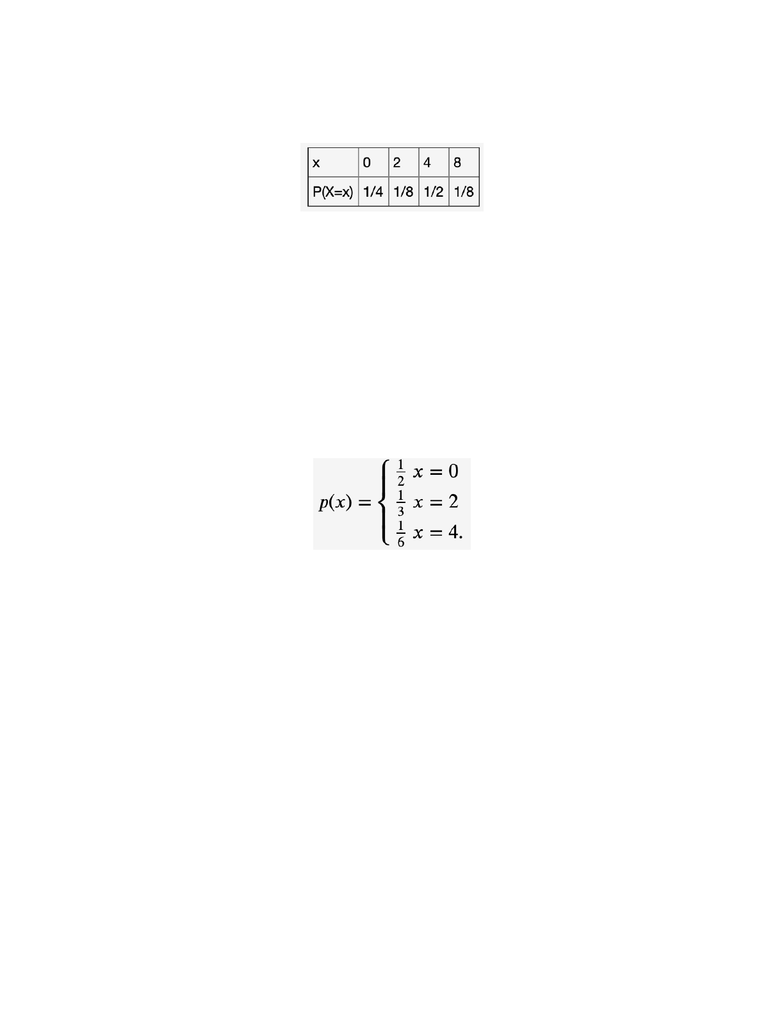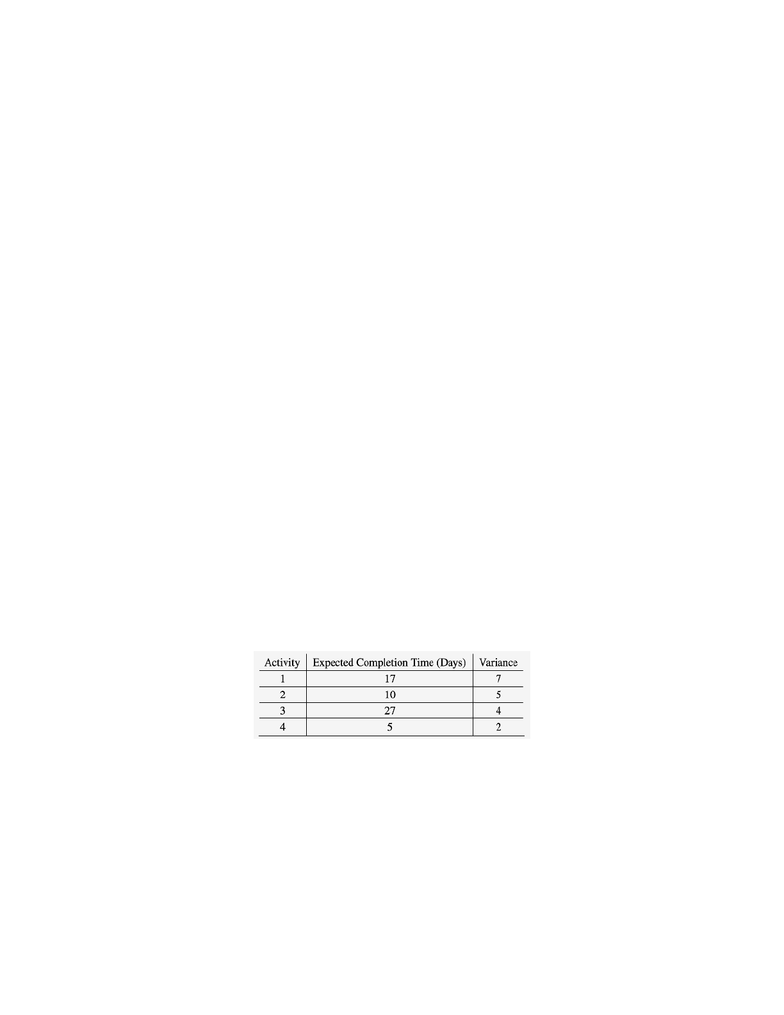# STAT 213 Chapter Notes - Chapter 3: Scotiabank Saddledome, Random Variable, Standard Deviation

50 views20 pagesChapter 3: Discrete Random Variables
1. The discrete random variable X has a probability distribution defined
below. What is the expectation of X?
2. The random variable X has mass function!given below. Find E(g(X)) where
g(x) = x^2 + x + 1
3. Three Toronto Maple Leaf fans attend a Flames-Leafs game in the
Saddledome. The probability that the first fan will wear their "Leafs"
jersey is 0.68. The probability that the second fan will wear their
"Leafs" jersey is 0.65. The probability that the third fan will not wear
their "Leafs" jersey is 0.56. Let X be a random variable which measures
how many of the three Leaf fans mentioned are wearing their "Leafs"
jersey to this hockey game. Assuming that each "Leaf" fan mentioned wears
their "Leaf" jersey independently of each other, find the probability
distribution of X.!
Unlock document

This preview shows pages 1-3 of the document.
Unlock all 20 pages and 3 million more documents.Chapter 3: Discrete Random Variables
A. P(X = 0)
B. P(X = 1)
C. P(X = 2)
D. P(X = 3)
4. There are four activities along the critical path for a project. The
expected values and variances of the completion times of the activities
are listed below. Determine the expected value and variance of the
completion time of the project.
A. Expected value of completion time of project:
Unlock document

This preview shows pages 1-3 of the document.
Unlock all 20 pages and 3 million more documents.Chapter 3: Discrete Random Variables
B. Variance of completion time of project:
5. The mean and standard deviation of a random variable x are 11 and 1,
respectively. Find the mean and standard deviation of the given random
variables:
A. y = x + 5
B. v = 7x
C. W = 7x + 5
6. Based on recent records, the manager of a car painting center has
determined the following probability distribution for the number of
customers per day. If the centre has the capacity to serve 2 customers
per day:
Unlock document

This preview shows pages 1-3 of the document.
Unlock all 20 pages and 3 million more documents.